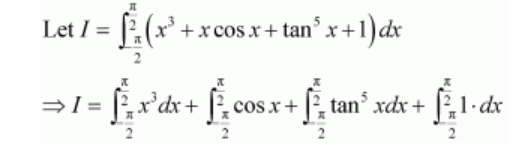# Show that

Question:

The value of $\int_{-\frac{\pi}{2}}^{\frac{\pi}{2}}\left(x^{3}+x \cos x+\tan ^{5} x+1\right) d x$ is

A. 0

B. 2

C. $\pi$

D. 1

Solution:It is known that if $f(x)$ is an even function, then $\int_{-a}^{a} f(x) d x=2 \int_{0}^{a} f(x) d x$ and

if f(x) is an odd function, then $\int_{-a}^{a} f(x) d x=0$

$I=0+0+0+2 \int_{0}^{\pi} 2^{2} 1 \cdot d x$

$=2[x]_{0}^{\frac{\pi}{2}}$

$=\frac{2 \pi}{2}$

$\pi$

Hence, the correct answer is C.